# ggpval

`ggpval` allows you to perform statistic tests and add the corresponding p-values to ggplots automatically. P-values can be presented numerically or as stars (e.g. , *). Alternatively, one can also make any text annotation between groups.

## Installation

``````# Install `ggpval` from CRAN:
install.packages("ggpval")

# You can install the lastest ggpval from github with:
# install.packages("devtools")
devtools::install_github("s6juncheng/ggpval")
``````

## Example

Simulate data with groups.

``````library(ggpval)
library(data.table)
library(ggplot2)
A <- rnorm(200, 0, 3)
B <- rnorm(200, 2, 4)
G <- rep(c("G1", "G2"), each = 100)
dt <- data.table(A, B, G)
dt <- melt(dt, id.vars = "G")
``````

## A trivial boxplot example

Give the group pairs you want to compare in `pairs`.

``````plt <- ggplot(dt, aes(variable, value)) +
geom_boxplot() +
geom_jitter()

add_pval(plt, pairs = list(c(1, 2)))
``````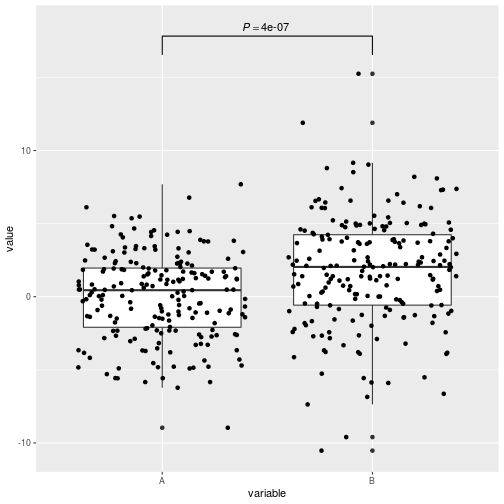## Boxplot with facets

``````plt <- ggplot(dt, aes(variable, value)) +
geom_boxplot() +
geom_jitter() +
facet_wrap(~G)
add_pval(plt, pairs = list(c(1, 2)))
``````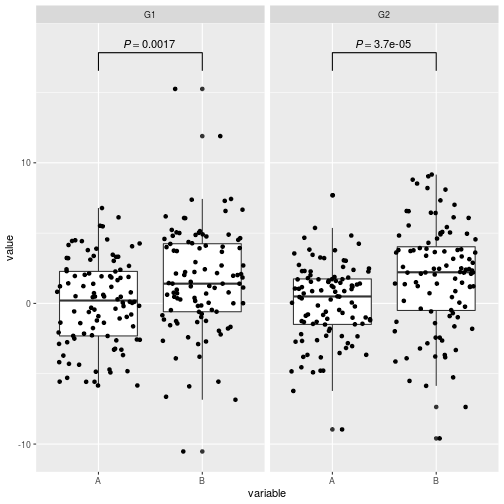## Bar plot

`ggpval` tries to infer the column which contains the data to do statistical testing. In case this inference was wrong or not possible (for instance the raw data column was not mapped in ggplot object), you can specify the correct column name with `response=`.

``````dt[, mu := mean(value),
by = c("G", "variable")]

dt[, se := sd(value) / .N,
by = c("G", "variable")]

plt_bar <- ggplot(dt, aes(x=variable, y=mu, fill = variable)) +
geom_bar(stat = "identity", position = 'dodge') +
geom_errorbar(aes(ymin=mu-se, ymax=mu+se),
width = .2) +
facet_wrap(~G)

add_pval(plt_bar, pairs = list(c(1, 2)), response = 'value')
``````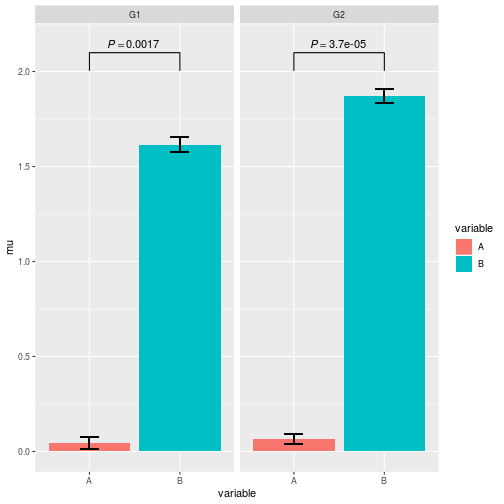Additional arguments for statistical function can also be directly specified.

``````add_pval(plt_bar, pairs = list(c(1, 2)),
test = 't.test',
alternative = "less",
response = 'value')
``````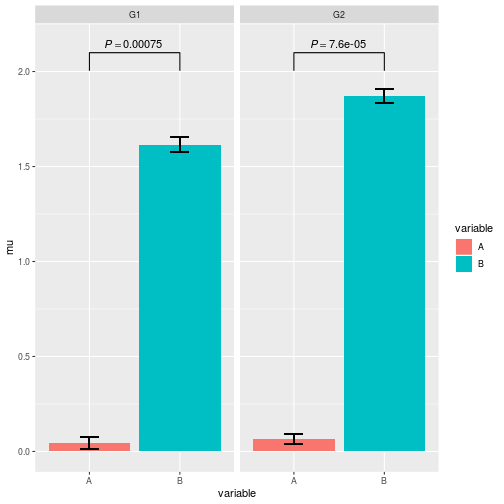## Annotate your plot

``````add_pval(plt, pairs = list(c(1, 2)), annotation = "Awesome")
``````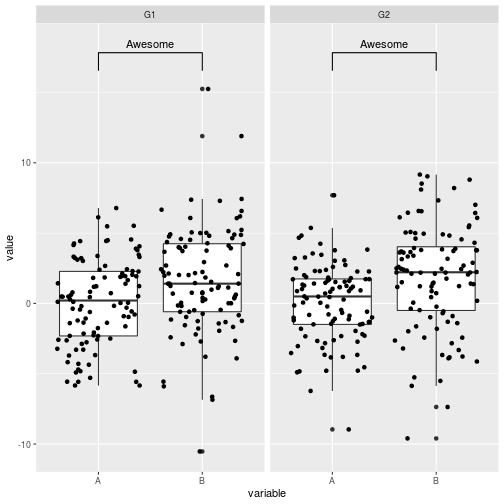In case you to want give different annotations to each facets, provide your annotation as a list

``````add_pval(plt, pairs = list(c(1, 2)), annotation = list("Awesome1", "Awesome2"))
``````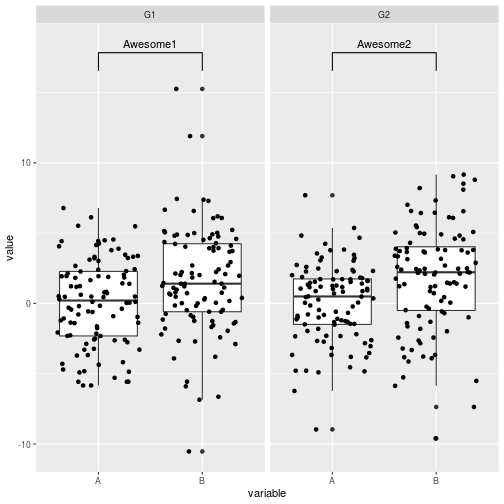## Bugs and issues

Please report bugs and issues on github issue page: https://github.com/s6juncheng/ggpval/issues. Contributions are welcome.

## Acknowledgement

Thanks to Vicente Yépez for testing and helping with improvement of the package.